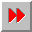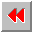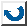EMA-XPS Online

DEFINE-CONSTRAINT

```DEFINE-CONSTRAINT
=================

syntax: (define-constraint <constraint-name> OF
<constraint-pattern-name>
[:DOCUMENTATION <constr.doc.>]
[:EXPLANATION <expl-expr.>]
[FOR-EACH
(<bab-var> <bab-lisp-expression>)]
WITH <simple-predication>+)

Warning: The explanation facility is not sup-
ported, hence :documentation and
:explanation are ignored.

With a constraint you define expressions for
the parameters of the constraint-pattern. In the
constraint-pattern is defined, how to link
certain values. The constraint defines
attribut-values of one or more instances.

FOR-EACH expects a list with a variable which is
followed by a lisp-expression. The lisp-expression
has to evaluate to a list with valid conditions.
For example: With the FOR-EACH declaration it is
possible to make a constraint for every instance
of a certain frame.

WITH expects a simple predication. The predications
in the WITH-clauses must not be based upon each other.
There must exist exactly one WITH-clause for every
parameter of the pattern.

example: >(define-constraint-pattern
calculate-real-costs)
(_real-costs _country-wishes)
:documentation "calculates the
costs for a visit of the countries
_country-wishes."
RULES
(KNOWN (_country-wishes)
THEN (is _real-costs (calculate-client-
costs _country-wishes))))
>(define-constraint calculate-costs-for-
client
OF calculate-real-costs
FOR-EACH (a-client (instances-of
'client)))
WITH
[complete-costs a-client _real-costs]
[destinations a-client _country-wishes])

```EMA-XPS Online